# 一、业务介绍

2016年1月22日，中国互联网络信息中心(CNNIC)发布的第37次《中国互联网络发展状况统计报告》显示，截至2015年12月，我国网络购物用户规模达到4.13亿，较2014年底增加5183万，增长率为14.3%，与此同时，手机网络购物用户规模增长迅速，达到3.40亿，手机网络购物的使用比例由42.4%提升至54.8%，随之而来的是网民在各种网络平台上所发表的购物观点、意见等的激增，这些评论文本包含了消费群体对所购买的商品或者服务的情感态度等信息，反映了用户通过互联网对产品各方面发表的看法，对电子商务平台销售者以及个体消费者都有重要的分析价值。Deloitte Consumer Products Group调查显示，有67%的网民会浏览在线评论，其中82%认为在线评论影响了他们的购买决策，可见，在线评论引发的电子口碑已不容小觑，通过评论分析，商家能够了解市场对产品的看法，发现与竞争对手的差异，为产品改进、价格优化等提供有价值信息。具体来讲，透过商品评论，销售者可以直接了解到当前出售产品的评论热点、提取产品优势和不足、挖掘顾客建议，甚至提前预测顾客需求，达到指导生产服务的目的；另外与商家的促销信息相比，在线评论具有独立性、非商业性，因此深得用户信赖，与此同时，由于缺少线下体验，更多的用户倾向于先看评论，后做决策，通过查看商品评价，消费者可以迅速了解到其他顾客对商品的评价、锁定关注属性的相关评价，支持消费决策。所以说，电子商务评论文本蕴含了顾客产品评分、销售数据等无法涵盖的重要信息，对评论文本的归纳与挖掘可以实现相关信息的提取，进而为产品经营乃至产品购买提供一定的决策支持1

# 二、数据描述

Paperwhite 902 42 32 73 153 602
Voyage 1633 94 44 147 331 1017
Kindle 2942 107 84 218 607 1926

# 三、数据建模

## （一）描述性分析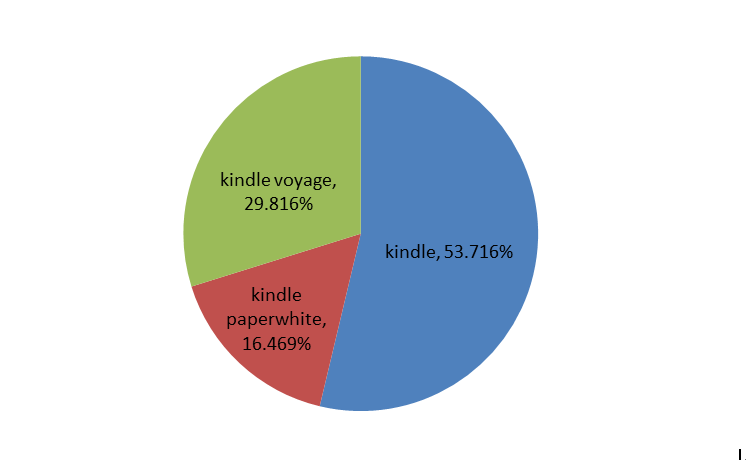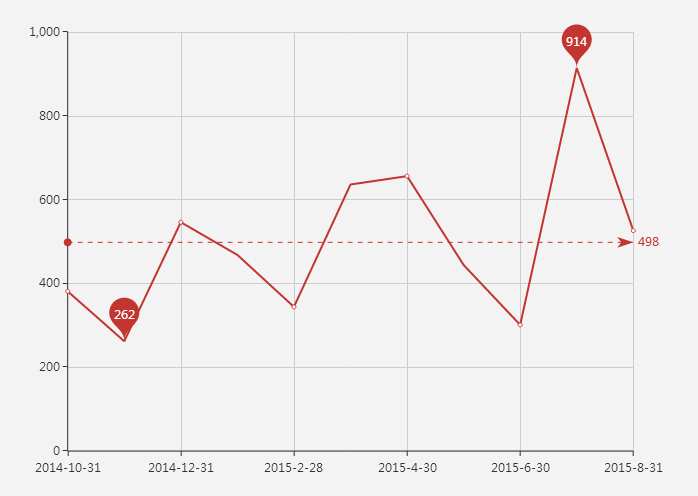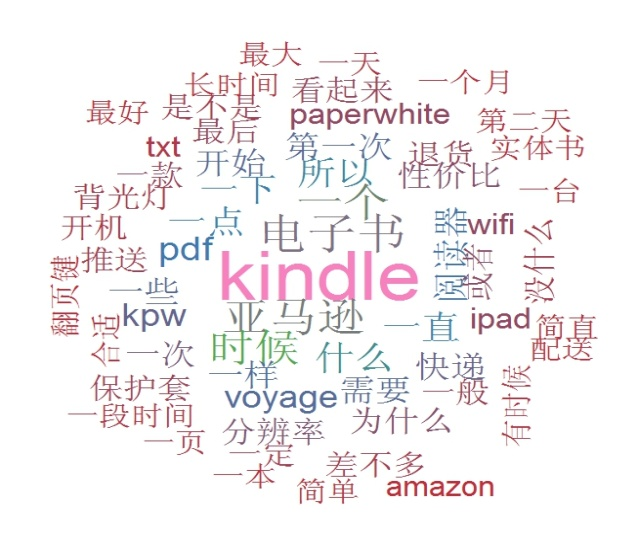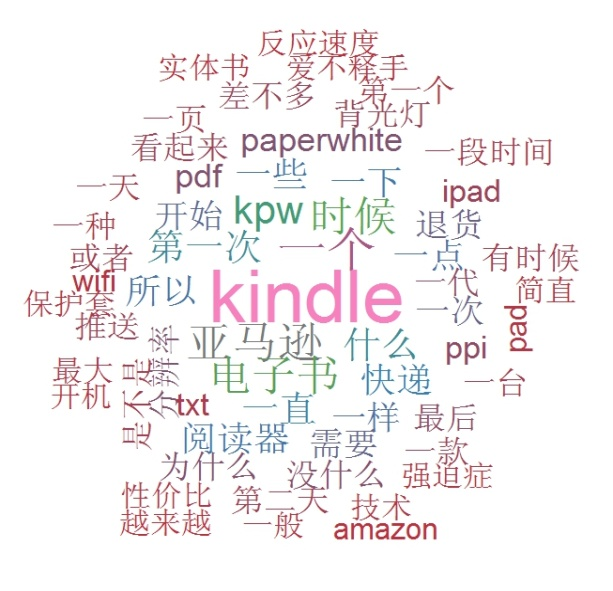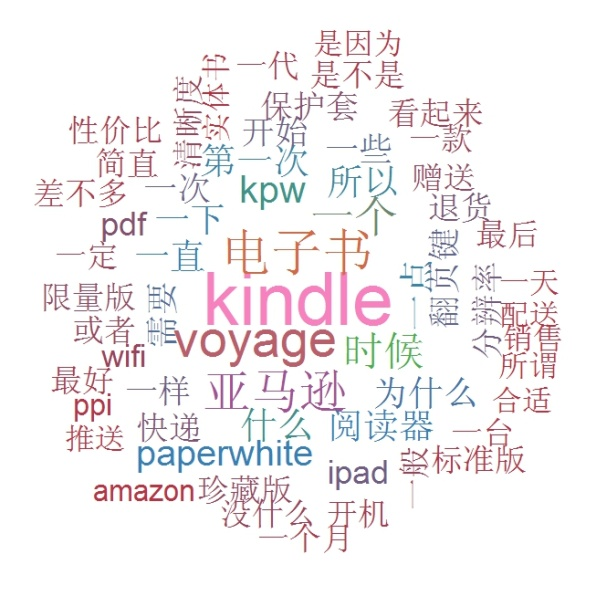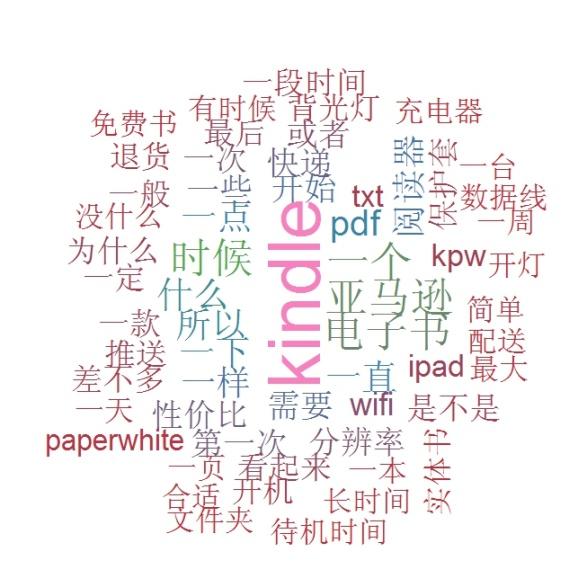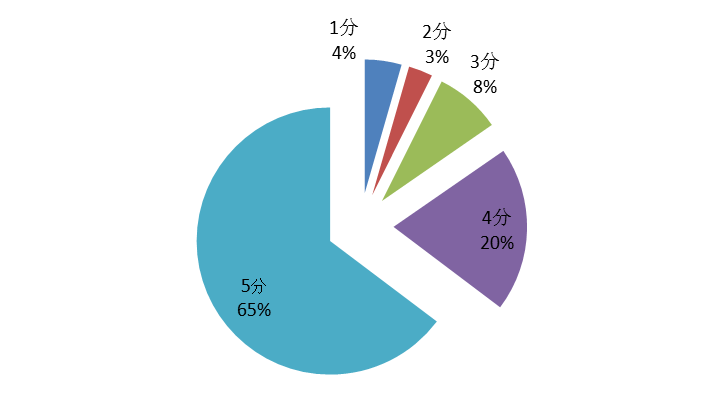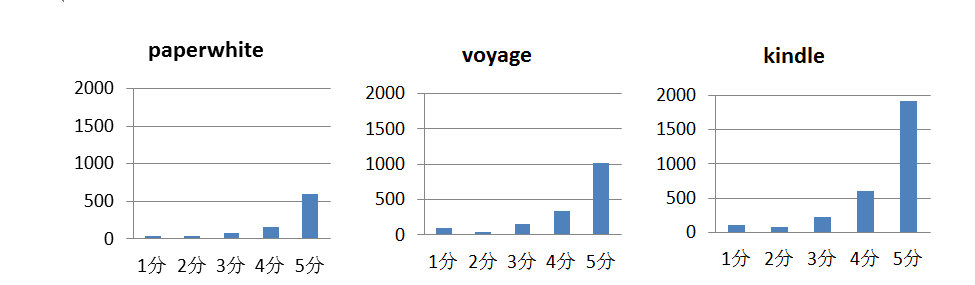## （二）模型设定

### （1）文本结构化处理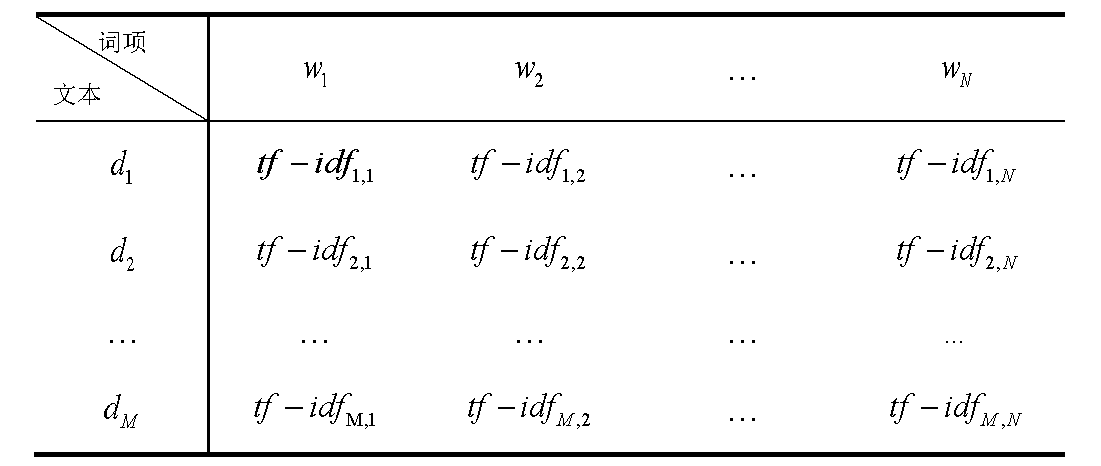$$tf_{ij} = \frac{n_ij}{\sum_{j=1}^Nn_{i,j}} \quad \quad(1)$$

$n_{i,j}$表示词项$\omega_j$ 在文本$d_i$中的频数，$\sum_{j=1}^Nn_{i,j}$则为文本$d_i$的总词数；

$$idf_j=log \frac{|D|}{|\{i : \omega_j \in d_i\}|} \quad \quad(2)$$

$|D|$为文本集合 中的总文本数， $|\{I:w_j \in d_i \}|$表示包含词项 $w_j$的总文本数，由于矩阵的稀疏性，$|\{I:w_j \in d_i \}|$ 可能趋近于零，故一般采用$idf_i=log\frac{|D|}{1+|\{i:w_j \in d_i \}|}$ 的计算形式。

### （2）聚类模型设定

$$p_k=\frac{\pi_k}{\sqrt{2\pi} \Pi_{j=1}^N \sigma_j} exp(-\sum_{j=1}^N\frac{(tf-idf_j^*-\mu_{kj})^2}{2\sigma_j^2}),k=1,\cdots,K （3）$$

$d^*$将被归类于$p_k$最大的那一类。

$\Theta = \{\sigma_j^2,\pi_k,\mu_{kj},k=1,\cdots,K;j=1,\cdots,N\}$作为包含所有参数的集合，给定数据$d_1,\cdots,d_n$，对数似然函数为：

$$l_0(\Theta)=\sum_{i=1}^Mlog(\sum_{k=1}^K \pi_kN_k(d_i;\mu_k,\Sigma_k) （4）$$

$$l_p(\Theta)=l_0(\Theta)-J(\Omega)\quad \quad（5）$$

$$\Omega = \{\mu_{kj},k=1,\cdots,K;j=1,\cdots,p \} \quad \quad （6）$$

$$J(\Omega)=\lambda \sum_{k=1}^K\sum_{j=1}^N|\mu_{kj}| \quad \quad （7）$$

$$l_p(\Theta) = \sum_{i=1}^M \sum_{k=1}^M \tau_{ik}(log\pi_{k} + logN_k(N_i; \mu_k,\Sigma)) – J(\Omega) \quad \quad（8）$$

## （三）模型估计结果

1 11 闪屏 4 11 价格偏高
2 5 免费书少 5 12 不伤眼
3 11 有亮点 6 7

$$p(i,j)=\frac{\max\limits_i (n(i,j))}{\sum_{i=1}^In(i,j)} \quad \quad (9)$$

$$P=\sum_{j=1}^J \frac{\sum_{i=1}^In(i,j)}{n}p(i,j) \quad \quad (10)$$

$$= \sum_{j=1}^J\frac{\max\limits_i (n(i,j))}{n}$$

$$precision(i,j) =\frac{n(i,j)}{\sum_{i=1}^I n(i,j)} \quad \quad（11）$$

$$recall(i,j) = \frac{n(i,j)}{n_i} \quad \quad （12）$$

$$f(i,j)=\frac{2 \times precision(i,j) \times recall(i,j)}{recision(i,j) + recall(i,j) }（13）$$

$$F=\sum_{i=1}^I\frac{n_i}{n}max(f(i,j)) \quad \quad（14）$$

 K-means欧式距离 K-means余弦距离 惩罚GMM 聚类纯度 F值 – – 35.09% 0.35 71.93% 0.62

# 四、业务实施

 类标签 评论数 评论语料 喜欢 116 挺喜欢的，有一些小缺陷，但也没有其他评论说的那么恐怖 女朋友是很爱看书的类型 但是携带起来不太方便 就给她买了kindle 她说很喜欢 手感也很好 不错的电子书阅读器，给女儿买的，看来她很喜欢 很喜欢，可以好好阅读了 很喜欢，感觉像宝藏一样的，去哪都可以带着它，可以利用很多碎片时间来看书，太阳底下也很清楚哦，喜欢读书的你们，赶快下手吧，你不会后悔的：） …… 翻页闪屏 184 似乎反应不是很灵敏, 要很长时间才能翻页. 另外, 没有纸制的说明书 之前使用kp2，确实有闪屏，但看书时就会被书的内容吸引，闪屏问题就会潜意识忽略了。kp3相比，性能提升了很多，很细腻，体验很好。培养看书习惯之良品 第一次用这电子阅读器，翻页效果一闪一闪的，实在受不了 总体还不错 四星是因为价格稍贵和无法避免的闪屏 背光很有用 亮度也可以调节 每次翻页都会闪两下  对于眼睛来说不是很舒服  但时间长了就习惯了 …… 感觉不错 200 第一次买kindle，刚好新出了一款，感觉还是不错的 用了一段时间，感觉还不错。也没碰到常见的商品问题。 不错，300ppi果然好多了啊 用来看书不错，反应速度有点迟钝 第一个发光不均匀，售后和快递服务都不错。拍照，技术确认，很快就给发了个新的。新的还不错，使用再看看 ……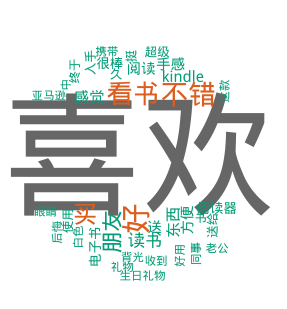# 五、总结与讨论

1. Dave K, Lawrence S, Pennock D M. Mining the peanut gallery: Opinion extraction and semantic classification of product reviews[C]//Proceedings of the 12th international conference on World Wide Web. ACM, 2003: 519-528.
2. 王和勇, 蓝金炯. 面向海量高维数据的文本主题发现[J]. 情报杂志, 2015, 34(11),162-167.
3. 张亮, 李敏强. 一种有限混合模型对无监督文本聚类的广义方法[J]. 模式识别与人工智能, 2007, 20(5),698-703.
4. Pan W, Shen X. Penalized model-based clustering with application to variable selection[J]. The Journal of Machine Learning Research, 2007, 8, 1145-1164.
5. Willett P. Document clustering using an inverted file approach[J]. Journal of Information Science, 1980, 2(5), 223-231.
6. Dempster A P, Laird N M, Rubin D B. Maximum likelihood from incomplete data via the EM algorithm[J]. Journal of the royal statistical society. Series B (Statistical Methodology), 1977, 1-38.
7. Maugis-Rabusseau C, Michel B. Adaptive density estimation for clustering with Gaussian mixtures[J]. ESAIM: Probability and Statistics, 2013, 17, 698-724.
8. Hatagami Y, Matsuka T. Text mining with an augmented version of the bisecting k-means algorithm[C]//Neural Information Processing. Springer Berlin Heidelberg, 2009, 352-359.
9. Yao M, Pi D, Cong X. Chinese text clustering algorithm based k-means[J]. Physics Procedia, 2012, 33: 301-307.
10. Zhao P, Rocha G, Yu B. Grouped and hierarchical model selection through composite absolute penalties[J]. Department of Statistics, UC Berkeley, Tech. Rep, 2006, 703.